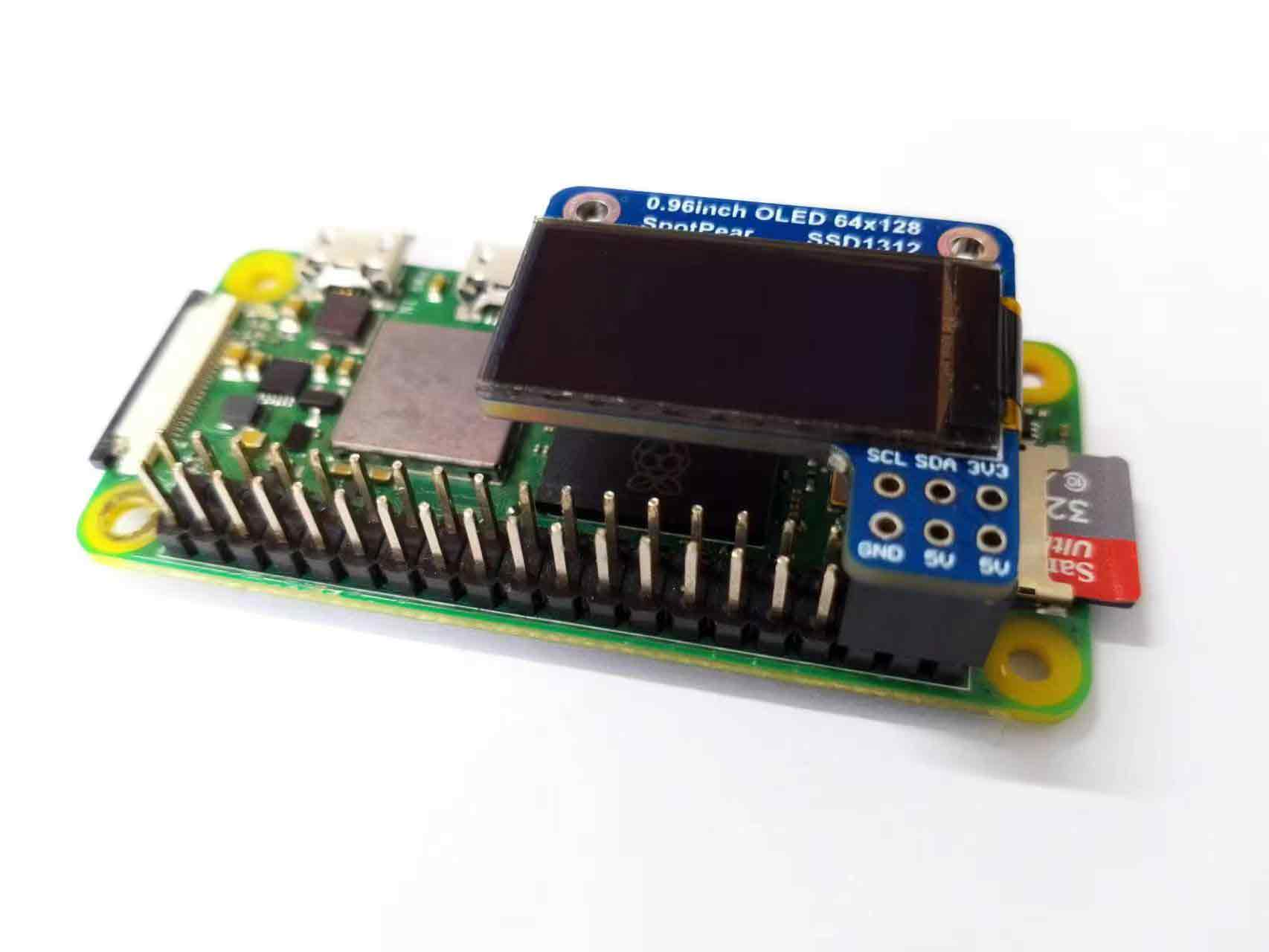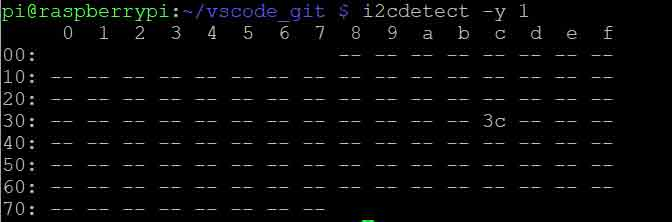•• (0)
• Account
• sales

+86-0755-88291180

# Raspberry Pi 0.96 64x128 SSD1312 OLED User Guide

## 【Preparatory work】

Before you begin, you need the following items:

[] Raspberry Pi

[] SSD1312 OLED Screen -I2C display interface

[] Python

Hardware connectionRun I2C interface

Restart the Raspberry Pi after ensuring that the I2C interface is turned on.

You can use the following command to find the address of the I2C device connected to the raspberry pi:

sudo i2cdetect -y 1

The running result is as shown in the figure (the default i2c address of ssd1306oled is 0x3c).Install luma.oled library

Next, you need to install the luma.oled library on the raspberry pi. You can install in the terminal by the following command:

sudo pip3 install luma.oled

Test Python program

Before running the code, you need to create a new python file named SSD 1306 _ OLED _ 128x64 _ bad _ apple.py in Raspberry Pi (the file name can be customized).

Combine the above steps, and drive the SSD1312 OLED screen by using luma.oled library. (The driving principle of sd1312 is the same as that of ssd1306, except that the display of ssd1312 is mirrored by default, and the mirroring problem can be solved by adding code to modify the mirror register in the code.) The following is a complete Python program for driving the SSD1312 OLED screen on Raspberry Pi:

import os

import time

from PIL import Image, ImageSequence

from PIL import ImageFont, ImageDraw

from luma.core.interface.serial import i2c

from luma.core.render import canvas

from luma.oled.device import ssd1306

device = ssd1306(serial, width=128, height=64)

# Invert the view

device.command(0x00)  # The 0xA0 instruction is set to the default column address 0.

device.command(0xA0)  # 0xA7 instruction turns on pixel inversion.

# Return CPU temperature as a character string

def getCPUtemperature():

return(res.replace("temp=","").replace("'C\n",""))

# Return RAM information (unit=kb) in a list

# Index 0: total RAM

# Index 1: used RAM

# Index 2: free RAM

def getRAMinfo():

p = os.popen('free')

i = 0

while 1:

i = i + 1

if i==2:

return(line.split()[1:4])

# Return % of CPU used by user as a character string

def getCPUuse():

return(str(os.popen("top -n1 | awk '/Cpu\(s\):/ {print \$2}'").readline().strip()))

# Return information about disk space as a list (unit included)

# Index 0: total disk space

# Index 1: used disk space

# Index 2: remaining disk space

# Index 3: percentage of disk used

def getDiskSpace():

p = os.popen("df -h /")

i = 0

while 1:

i = i +1

if i==2:

return(line.split()[1:5])

def draw_text(text, width, height, fontsize):

font = ImageFont.truetype("arial.ttf", fontsize)

with canvas(device) as draw:

draw.rectangle(device.bounding_box, outline="white", fill="black")

# Calculate the width of the text using textlength() instead of textsize()

w = font.getlength(text)

# Calculate the height of the text by subtracting the font descent from the font ascent

ascent, descent = font.getmetrics()

h = ascent - descent

# Calculate the position of the text

x = (width - w) // 2

y = (height - h) // 2

# Draw the text using the new width and height values

draw.text((10, 1), text, font=font, fill="white")

def image_gif():

# Display each image with an idea

for frame in frames:

device.display(frame)

time.sleep(0.01)

def computer_resources(flag):

while not flag.is_set():

# CPU informatiom

CPU_use = getCPUuse()

CPU_tmp = getCPUtemperature()

# RAM information

# Output is in kb, here I convert it in Mb for readability

RAM_stats = getRAMinfo()

RAM_total = round(int(RAM_stats) / 1000,1)

RAM_used = round(int(RAM_stats) / 1000,1)

RAM_free = round(int(RAM_stats) / 1000,1)

# Disk information

DISK_stats = getDiskSpace()

DISK_total = DISK_stats

DISK_used = DISK_stats

DISK_perc = DISK_stats

#

status_text = "CPU_use:  "+CPU_use+"\nCPU_tmp:  "+CPU_tmp+"\nRAM_used:  "+str(RAM_used)+"\nDISK_used:  "+DISK_used

draw_text(status_text, device.width, device.height, 12)

if __name__ == '__main__':

# Open a GIF file and create an Image object for each frame

frames = []

for frame in ImageSequence.Iterator(img):

frames.append(frame.convert("1").resize((128,64)))

# Create a threading.Event() object for synchronization

# Create a thread to compute system resources usage

while True:

# Show some text and wait for 2 seconds

draw_text("        Spotpear\nhttp://www.spotpear.cn\nhttp://www.spotpear.com\n", device.width, device.height, 12)

time.sleep(2)

#Start the thread to compute system resources usage,wait for 5 seconds

t.start()

time.sleep(5)

# Set the flag to stop the thread

flag.set()

# Wait for the thread to terminate

t.join()

# Clear the flag and create a new thread for next loop

flag.clear()

time.sleep(2)

# Display Bad Apple animation as GIF images

image_gif()

Note that we used the address "0x3C" in the i2c object, which is the default SSD1312 OLED screen address. If you are using other types of i2c devices, use the command sudo i2cdetect -y 1 to find its address and replace it with the address in the I2C object.

This program realizes the display effect of gif, so it must have a corresponding gif file, otherwise the program can't run normally.

How to convert a video into a gif refer to this tutorial: Converting MP4 video into gif

Program execution effect

Input the above code into python file, save and exit, and input the following code in Raspberry Pi terminal to execute the program: